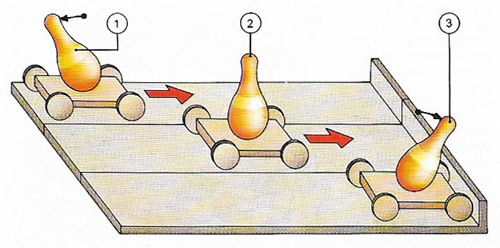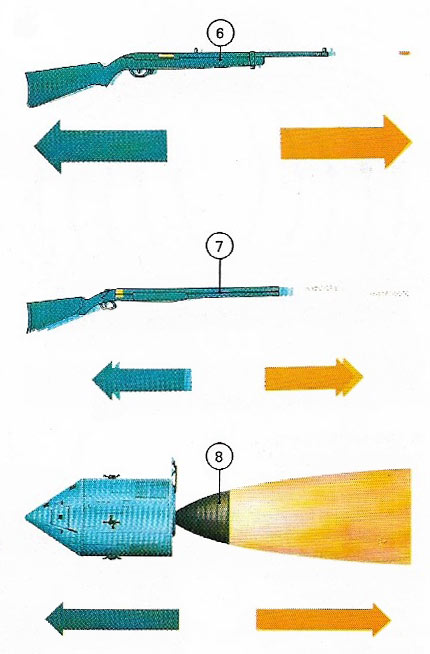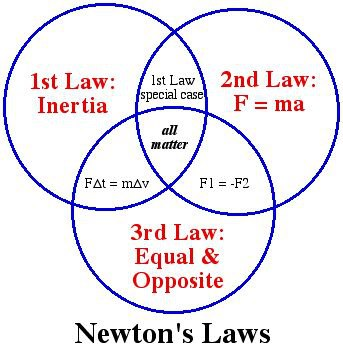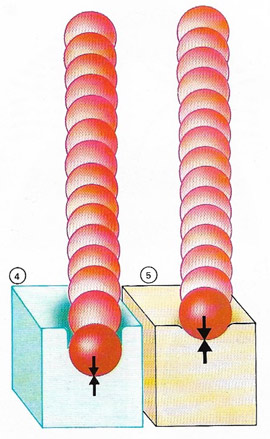A# Newton's laws of motionFig 1. Newton's first law of motion describes inertial effects. An object resists being from rest by toppling backwards (1), although moving steadily it is undisturbed, as if at rest (2). When stopped it resists slowing and tends to continue moving (3).Fig 3. Newton's third law states there is an equal and opposite reaction to every force. A rifle recoils when fired (6), although the bullet's velocity is much greater. Successive firings cause successive recoils (7). A rocket ejects gas and moves forwards (8) because of reaction.Fig 4. An experiment designed to prove the validity of the third law is shown in this early 18th century book on Newton's laws. A metal globe emits a jet of stream in one direction and causes the "engine" to react by moving in the opposite direction.Fig 5. Newton's laws of motion as a Venn diagram.

Newton's laws of motion are three fundamental laws describing the dynamic behavior of objects (see dynamics), first published by Isaac Newton in his great work Principia in 1687. They are as follows:

## First law of motion

A body will remain in a state of rest or uniform motion unless acted upon by a net external force (Fig 1). The first law is also called the "law of inertia" because it enshrines the concept of inertia as developed by Galileo.

An example of the first law is provided by the motion of a car and its passengers. Once a car is in motion, both it and its passengers carry on unless acted on by a force – such as a braking force. A head-on collision may stop the car, but the inertia of the passengers will cause them to fly forwards from their seats. They may be thrown against the wind shield unless held in place by safety belts on air bags, which exert a restraining force.

As a result of this law, it is apparent that the greater the applied force on an object the greater its change of velocity. Velocity is merely speed in a certain direction, and change of velocity in a given time is called acceleration.

## Second law of motion

The net force on a body is equal to the product of the body's mass and its acceleration (i.e., the rate of change of momentum). Acceleration and force are vectors. In the second law, the directions of the force vector and the acceleration vector are the same.Fig 2. A ball falling on to a soft material (4) sinks deeper than into a harder one (5) because the deceleration force is smaller.

## Third law of motion

When two bodies interact, the force on the first due to the second is equal and opposite to the force on the second due to the first. In other words, action and reaction are equal and opposite (Fig 3).

### D'Alembert's principle

D'Alembert's principle is the observation that Newton's third law of motion (that to every action there is an equal and opposite reaction) applies not only to systems in static equilibrium but also to those in which at least one component is free to move. Thus when a force F is applied to an unconstrained body of mass m endowing it with acceleration a, there is an equal and opposite inertial reaction -ma.